Symmetric Solid Objects HomePage

# On Symmetric Structures within Symmetric Solid Objects

### Summary and Key Findings :

#### Key Finding One :

• The 20-pieces Icosahedron is made-up-of 5 identical { Quad-Type Structures } ,

• with each { Quad-Type Structure } being in-itself-and-by-itself [ quad symmetric ] .

( see Section XXV / Part VIII for details ) .

And there are two (2) different-and-distinct ways to split the 20 pieces of the Icosahedron into 5 identical { Quad-Type Structures } .

#### Key Finding Two :

• Duality for [ Dodechedron vs. Icoshedron ] may be linked via the [ OHATS ( 120-Split ) Sphere ] :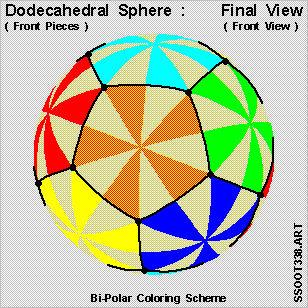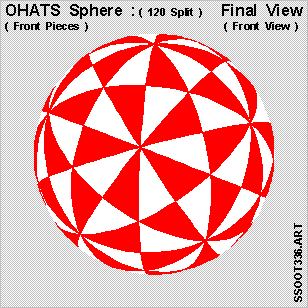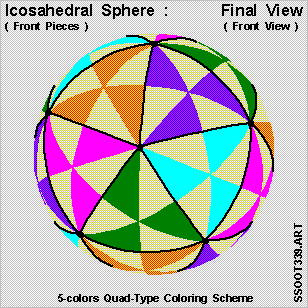#### Key Finding Three :

• This [ 5-colors configuration ] of the Icosahedron is [ quad-symmetric ] :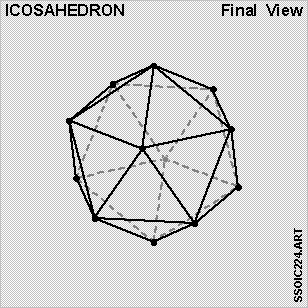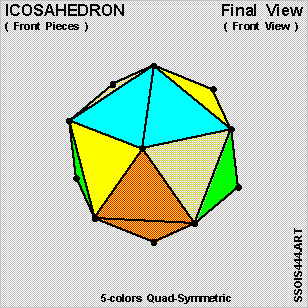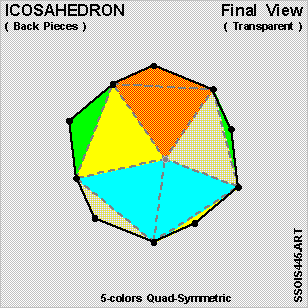#### Key Finding Four :

• This [ 4-colors configuration ] of the Icosahedron is [ 2-6-6-6-symmetric ] :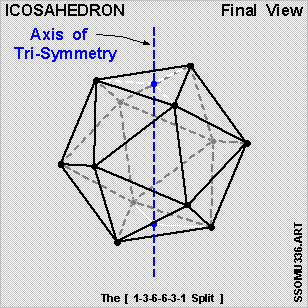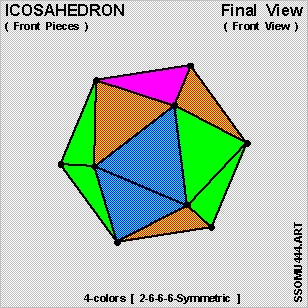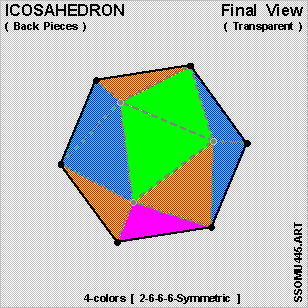#### Table of Content

Part I --- Introduction and Preliminaries Part II --- Symmetric Solid Object Basics Section I Introduction Section II A Special Note on the Notations Section III Basic Properties of the Tetrathedron Section IV Basic Properties of the Cube Section V Basic Properties of the Octohedron Section VI Basic Properties of the Dodecahedron Section VII Basic Properties of the Icosahedron Section VIII The Bi-Symmetric Nature of the Tetrahedron Section IX The Bi-Symmetric Nature of the Cube Section X The Tri-Symmetric Nature of the Cube Section XI The Bi-Symmetric Nature of the Octahedron Section XII The Quad-Symmetric Nature of the Octahedron Section XIII Brief Summary on the Tetrahedron / Cube / Octahedron Section XIV Bi-Polar Structures within the Dodecahedron Section XV The Bi-Symmetric Nature of the Dodecahedron Section XVI The Tri-Symmetric Nature of the Dodecahedron Section XVII The Quad-Symmetric Nature of the Dodecahedron Section XVIII The Hexa-Symmetric Nature of the Dodecahedron Section XIX Bi-Polar Structures within the Icosahedron Section XX The Bi-Symmetric Nature of the Icosahedron Section XXI The Quad-Symmetric Nature of the Icosahedron Section XXII The Penta-Symmetric Nature of the Icosahedron Section XXIII The Deci-Symmetric Nature of the Icosahedron Section XXIV The [ 2-6-6-6-Symmetry ] co-existing within the Icosahedron Section XXV [ Quad-Type Structures ] within the Icosahedron Section XXVI Defining Great-Circles , Great-Cricle-Planes and Great-Circle-Arcs Section XXVII The Tetrahedral Sphere ( 4-Split ) Section XXVIII The Quasi-Cubic Sphere ( 6-Split ) Section XXIX The Octahedral Sphere ( 8-Split ) Section XXX The Dodecahedral Sphere ( 12-Split ) Section XXXI The Icosahedral Sphere ( 20-Split ) Section XXXII Ratio-Of-Areas and the Egyptian Fractions Section XXXIII Duality - Tetrahedron vs. Tetrahedron Section XXXIV Duality Linkage via the [ TFS ( 24-Split) Sphere ] Section XXXV Duality - Cube vs. Octahedron Section XXXVI Duality Linkage via the [ FES ( 48-Split ) Sphere ] Section XXXVII Duality - Dodecahedron vs. Icosahedron Section XXXVIII Duality Linkage via the [ OHATS ( 120-Split ) Sphere ] Section XXXIX Identifying 2 [ Quad-Type Structures ] for Our Analysis Section XXXX A First Look at the 2 [ Quad-Type Structures ] Section XXXXI Rotational Orientation of the [ Curved Triangular Pieces ] Section XXXXII A Special Feature of the [ Quad-Type Coloring Schemes ] Section XXXXIII 5-Colors Combinatorics for the [ ALPHA Series ] Section XXXXIV 5-Colors Combinatorics for the [ OMEGA Series ] Section XXXXV Concluding Remarks